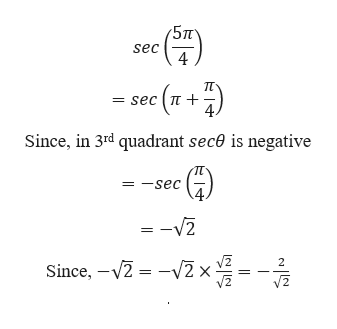# The question asks for the exact value of the trigonometric function at the given real number: sec(5pi/4)  I answered 2/(-(sqrt)2), but I would like to double check this answer...

Question

The question asks for the exact value of the trigonometric function at the given real number:

sec(5pi/4)  I answered 2/(-(sqrt)2), but I would like to double check this answer...

check_circleExpert Solution
Step 1

Given,

...help_outlineImage Transcriptionclose(5п sec 4 п - sec (n Since, in 3rd quadrant sec0 is negative -sec = =-V2 2 Since, -2 2x | fullscreen

### Want to see the full answer?

See Solution

#### Want to see this answer and more?

Solutions are written by subject experts who are available 24/7. Questions are typically answered within 1 hour*

See Solution
*Response times may vary by subject and question
Tagged in

### Trigonometric Ratios